• 12
•
• 5
•
• 2
•
•
•
19
Shares

# ØRSTED’S LAW AND OERSTED’S EXPERIMENT

### INTRODUCTION

We know that when an electric charge moves with a constant acceleration then it creates an electric field as well as a magnetic field. But how do we know that it creates a magnetic field? Where did the term magnetic field come from?

Well, in this article, we are going to discuss the interesting story of the discovery of the magnetic field. So let’s start…

## OERSTED’S EXPERIMENT

This was the day 21 April 1820, on this day, a Danish physicist Hans Christian Ørsted (1777–1851) has experimented and discovered the relationship between electricity and magnetism.

However, he had performed various experiments on the current-carrying conductors previously but this experiment is going to be different from all the previous experiments because in this experiment he unknowingly put a magnetic compass near a straight current-carrying conductor.

He put a straight wire vertically through a tabletop and put a magnetic compass near it. When he supplied steady DC current to the wire then he notices a small deflection in the compass needle. He became surprised and said in excitement what is this? See figure below:

When he turned off the current in the wire then again compass needle show deflections, which means turning on and off of electric current deflects the compass needle.

He became sure that something is emerging from the conductor which is invisible in nature when an electric current is given to the conductor.

He think about the magnetic compass which is kept near the wire. He thought that needle of the compass is made up of magnets and magnets only experience magnetic force when it exposes to the magnetic field. Means, this wire is creating magnetic field around itself. He has no idea that his thinking is going to bring revolutionary changes in the history of electromagnetism.

Further, he did this experiment in various ways and find several results regarding this experiment and these results became famous as Ørestd Law.

## OERSTED’S LAW

In electromagnetism, Ørestd Law also spelled as Orested’s Law is a physical law which states that-

Electric current produces magnetic field.

This law was discovered by the Danish physicist Hans Christian Ørsted (1777–1851) on 21 April 1820, when he noticed that the compass needle near to a current-carrying conductor turned so that the needle was perpendicular to the conductor.

Ørsted further investigated and found the physical law describing the magnetic field, now this physical law is known as Ørsted’s law.

It was the first found connection between electricity and magnetism, and the second law which links both to each other is Faraday’s law of induction. These two laws became part of the equations (Maxwell equation) that govern electromagnetism.

### OERSTED’S EXPERIMENT RESULTS

After performing a number of experiments and keen observations. Ørsted found these important results for a straight current-carrying conductor.

• A current-carrying conductor produces a magnetic field around it.
• The magnetic field lines also called lines of force encircle the current-carrying conductor. See figure 4 above, red concentric circles are the magnetic field lines.
• The magnetic field lines lie in a plane perpendicular to the conductor (i.e. at the right angle to each other).
• If the direction of flow of current is reversed then the direction of magnetic field lines is also reversed.
• The strength of the magnetic field is directly proportional to the current density.
• The strength of the magnetic field at a point is inversely proportional to the distance of that point from the current-carrying conductor.
• The magnetic field exists around the wire until the flow of current stops.

Some more results confirmed by the Ørsted experiment is as follows:

• If two parallel current-carrying conductors have the same current but opposite in direction then the resultant magnetic field will be zero. This is because both magnetic fields have opposite polarities and they repel each other.
• If two parallel current-carrying conductors have the same current and direction then the resultant magnetic field will be doubled. This is because both magnetic fields have the same polarity and they attract each other.
• The magnetic effect is directly proportional to the magnitude of the flowing current in the conductor.

### VECTOR FORM OF ØRSTED LAW

Ørsted law can be generalized to give the modern vector form. The mathematical statement of this law can be given as follows:

The line integral of the magnetic field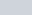around any closed curve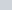is proportional to the total currentpassing through any surface bounded by the curve.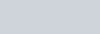Where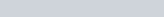is the magnetic constant and the direction of integration aroundis related to the direction of current in the conductor which is given by the right-hand rule.

This law can also be expressed in terms of the current density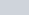through the surface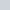instead of the total current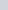through it.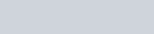whereis any surface spreaded in.

## SOME USEFUL INFORMATION REGARDING THIS LAW

Keep in mind that Ørsted’s law can only hold for DC steady currents, which means the current which don’t change with time. So, it is only applicable for DC electric circuits, where no capacitors or inductors are being used.

It fails for time-varying currents i.e alternating current by considering the case of a circuit in which a battery is charging a capacitor through a resistor.

## APPLICATIONS OF ØRSTED LAW

There are various applications of Ørestd Law. Ørsted experiment laid the foundation for various advanced electrical machinery. It is listed as one of the Maxwell equations. Some applications of Ørestd Law is given below:

• Electric Motors.
• Electric Transformers.
• Electric Generators and alternators.
• Headphones, microphones, and tap record players.
• Magnetometers
• Musical instruments such as electric guitar etc.
• Digital Microcontrollers and Microprocessors.

Watch this video for more understandings.

Stay tuned with Laws Of Nature for more useful and interesting content.